# STL - krishnaramb/cplusplus Wiki

### Introduction

• The STL consists of the iterator, container, algorithm, and function object parts of the standard library.

### Container Overview

• A container holds a sequence of objects. Containers can be categorized like this:

• Associative containers provide associative lookup based on a key

• Almost containers are sequences of elements that provide most, but not all, of the facilities of a container.

• The STL containers (the sequence and associative containers) are all resource handles with copy and move operations :rose:

• All operations on containers provide the basic guarantee to ensure that they interact properly with exception-based error handling :rose:

• Sequence Containers

• `vector<T,A>`
• A contiguously allocated sequence of `T`s; the default choice of container
• `list<T,A>`
• A doubly-linked list of `T`; use when you need to insert and delete elements without moving existing elements
• `forward_list<T,A>`
• A singly-linked list of `T`; ideal for empty and very short sequences
• `deque<T,A>`
• A double-ended queue of `T`; a cross between a vector and a list; slower than one or the other for most uses The `A` template argument is the allocator:punch:that the container uses to acquire and release memory for example,
``````template<typename T, typename A = allocator<T>>
class vector {
// ...
};
``````
• `A` is defaulted to `std::allocator<T>` which uses `operator new()`and `operator delete()` when it needs to acquire or release memory for its elements.:hibiscus::herb:

• These containers are defined in `<vector>`, `<list>`, and `<deque>`. The sequence containers are contiguously allocated (e.g., `vector`) or linked lists (e.g., `forward_list`) of elements of their `value_type` (`T` in the notation used above). A `deque` (pronounced ‘‘deck’’) is a mixture of linked-list and contiguous allocation.

• Unless you have a solid reason not to, use a vector. Note that vector provides operations for inserting and erasing (removing) elements, allowing a vector to grow and shrink as needed.

• When inserting and erasing elements of a vector, elements may be moved :punch:. In contrast, elements of a list or an associative container do not move when new elements are inserted or other elements are erased.:punch:

• Ordered Associative Containers

• map
• multimap
• set
• multiset
• All these Ordered Associative containers are usually implemented as balanced binary trees (usually red-black trees). :rose::revolving_hearts:

### Container Representation

• In addition to what is needed to manipulate elements, such a ‘‘handle’’ will hold an allocator
• For a `vector`, the element data structure is most likely an array: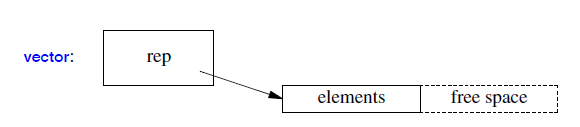• The `vector` will hold a pointer to an array of elements, the number of elements, and the capacity (the number of allocated, currently unused slots) or equivalent
• A `list` is most likely represented by a sequence of links pointing to the elements and the number of elements: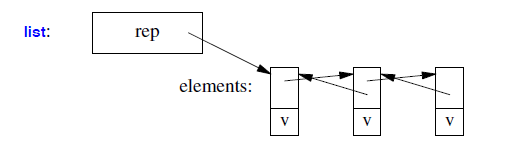• A `forward_list` is most likely represented by a sequence of links pointing to the elements: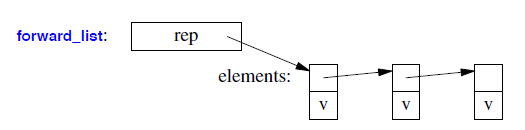### Size and Capacity

The size is the number of elements in the container; the capacity is the number of elements that a container can hold before allocating more memory

• When changing the size or the capacity, the elements may be moved to new storage locations. That implies that iterators (and pointers and references) to elements may become invalid (i.e., point to the old element locations).
• An iterator to an element of an associative container (e.g., a `map`) is only invalidated if the element to which it points is removed from the container (`erase()`d)
• To contrast, an iterator to an element of a sequence container (e.g., a `vector`) is invalidated if the elements are relocated (e.g., by a `resize()`, `reserve()`, or `push_back()`) or if the element to which it points is moved within the container (e.g., by an `erase()` or `insert()` of an element with a lower index).
• It is tempting to assume that `reserve()` improves performance, but the standard growth strategies for vector are so effective that performance is rarely a good reason to use `reserve()`. Instead, see `reserve()` as a way of increasing the predictability of performance and for avoiding invalidation of iterators.

### Iterators

• A container can be viewed as a sequence either in the order defined by the containers iterator or in the reverse order.
• For an associative container, the order is based on the containers comparison criterion (by default `<`)
Iterators description
`p=c.end()` p points to one-past-last element of c
`cp=c.cbegin()` p points to constant first element of c
`p=c.cend()` p points to constant one-past-last element of c
`p=c.rbegin()` p points to first element of reverse sequence of c
`p=c.rend()` p points to one-past-last element of reverse sequence of c
`p=c.crbegin()` p points to constant first element of reverse sequence of c
`p=c.crend()` p points to constant one-past-last element of reverse sequence of c
• The most common form of iteration over elements is to traverse a container from its beginning to its end. The simplest way of doing that is by a range-`for` which implicitly uses `begin()` and `end()`. For example:
``````for (auto& x : v) // implicit use of v.begin() and v.end()
cout << x << '\n';
``````
• When we need to know the position of an element in a container or if we need to refer to more than one element at a time, we use iterators directly.
• In such cases, `auto` is useful to minimize source code size and eliminate opportunities for typos. For example, assuming a random-access iterator:
``````for (auto p = v.begin(); p!=end(); ++p) {
if (p!=v.begin() && ∗(p−1)==∗p)
cout << "duplicate " << ∗p << '\n';
}
``````
• When we don’t need to modify elements, `cbegin()` and `cend()` are appropriate. That is, I should have written:
``````for (auto p = v.cbegin(); p!=cend(); ++p) { // use const iterators
if (p!=v.cbegin() && ∗(p−1)==∗p)
cout << "duplicate " << ∗p << '\n';
}
``````

### `vector`

The `vector`’s template argument and member types are defined like this:

``````template<typename T, typename Allocator = allocator<T>>
class vector {
public:
using reference = value_type&;
using const_reference = const value_type&;
using iterator = /* implementation-defined */;
using const_iterator = /* implementation-defined */;
using size_type = /* implementation-defined */;
using difference_type = /* implementation-defined */;
using value_type = T;
using allocator_type = Allocator;
using pointer = typename allocator_traits<Allocator>::pointer;
using const_pointer = typename allocator_traits<Allocator>::const_pointer;
using reverse_iterator = std::reverse_iterator<iterator>;
using const_reverse_iterator = std::reverse_iterator<const_iterator>;
// ...
};
``````

### vector and Growth

Consider the layout of a vector object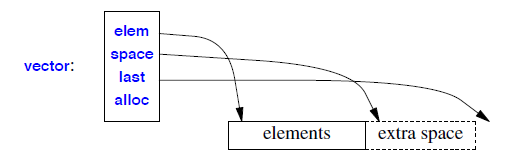• The use of both a size (number of elements) and a capacity (the number of available slots for elements without reallocation) makes growth through `push_back()` reasonably efficient: there is not an allocation operation each time we add an element, but only every time we exceed capacity:punch::heart:

### Different ways to initialize a vector 👊

• Initializing by pushing values one by one

``````// Create an empty vector
vector<int> vect;

vect.push_back(10);
vect.push_back(20);
vect.push_back(30);
``````
• Specifying size and initializing all values 🌺 🍀

``````int n = 3;
// Create a vector of size n with
// all values as 10.
vector<int> vect(n, 10);

for (int x : vect)
cout << x << " ";

``````
• Initializing like arrays

``````#include <bits/stdc++.h>
using namespace std;

int main()
{
vector<int> vect{ 10, 20, 30 }; //initializer list

for (int x : vect)
cout << x << " ";

return 0;
}
``````
• Initializing from another vector
``````// CPP program to initialize a vector from
// another vector.
#include <bits/stdc++.h>
using namespace std;

int main()
{
vector<int> vect1{ 10, 20, 30 };
vector<int> vect2(vect1.begin(), vect1.end());// note range is [ )
// whenever the range is mentioned, either in insert() or any others it
//  is [ ). i.e. the second value of the range is not inclusive
for (int x : vect2)
cout << x << " ";

return 0;
}
``````

👊 You cannot do

``````vector<string> name(5); //error in these 2 lines
vector<int> val(5,0);
``````

in a class outside of a method. 👊 It will throw out compilation error `expected identifier before numeric constant`.

You can initialize the data members at the point of declaration, but not with `()` brackets: :rose:

``````class Foo {
vector<string> name = vector<string>(5);
vector<int> val{vector<int>(5,0)};
};
``````

👊 Below code also throws out error due to the same reason

``````class Solution {
private:
vector<vector<bool>> visited((300, vector<bool>(300, false));
int n;
int m;
public:

``````

Instead, if we do the following,it works. 🌹

``````class Solution {
private:
vector<vector<bool>> visited{(300, vector<bool>(300, false))};
int n;
int m;
public:

``````

### How to initialize a 2D vector 👊 👊

• we can initialize 2D vector of N rows and M column, with a value 0 as below using a constructor🌹 💞
``````vector<vector<int>> v; // creates an empty two dimensional vector of ints.
``````
``````vector<vector<int>> v(3); // creates a 3 vector of empty vector
``````
``````vector<vector<int>> matrix(ROW, vector<int>(COL, default_value));
``````
`````` //if the default value is not given, it is filled with 0s
vector<vector<int>>matrix(ROW, vector<int>(COL))
``````
``````#include<bits/stdc++.h>
using namespace std;

int main(){
int n = 3; //no of rows
int m = 4;  //no of cols

// Create a vector containing n
//vectors of size m.
vector<vector<int> > vec( n , vector<int> (m, 0));

for (int i = 0; i < n; i++) {
for (int j = 0; j < m; j++){
cout<< vec[i][j]<< " ";
}
cout<< "\n";
}

return 0;
}
``````

### const vector and const iterators

• For the function like below, you need to use the const_iterator
``````int main()
{
vector<int> vect{1,3,4,4};
vect = 30; //vect is the l-value
vector<int>::iterator iter1 = vect.begin();
*iter1 = 20; // you can change the value using iter1 variable

const vector<int> cvect{1,3,4,4};
// cvect = 30; //Illegal: cvect is r-value and not the modifiable l-value
// vector<int>::iterator iter = cvect.begin();  //Illegal: you can't assign read only
// memory pointer to read/write pointer

vector<int>::const_iterator iter; // you can have it uninitialized because variable itself
// is not const, only memory pointed by it is constant

iter = cvect.begin();
// *iter = 20; //Illegal: iter is constant and you can't change the value

// you can have const_iterator for non-const vector as well
vector<int>::const_iterator citer = vect.begin();
// now you can't change the value of vect using citer variable
// *citer = 20; //Illegal: citer points to read only memory

// vector<int>::iterator iter2 = vect.cbegin(); // Illegal: cbegin() returns read-only iterator
vector<int>::const_iterator iter2 = vect.cbegin(); // Illegal: cbegin() returns read-only iterator

// since cvect is already const, there is no difference if you did cbegin() or begin().
// In both case, it will give you read-only memory location pointer

// vector<int> iter3 = cvect.cbegin(); // Illegal even if cvect is not const

}

``````

Do you need to have cbegin() when you already have const vector??

### How to insert element in vector at specific position

Vector provides different overloaded version of member function insert() , to insert one or more elements in between existing elements.

### Inserting a single element at specific position in vector

``````iterator insert (const_iterator pos, const value_type& val);
``````
``````std::vector<int> vecOfNums { 1, 4, 5, 22, 33, 2, 11, 89, 49 };
// Create Iterator pointing to 4th Position
auto itPos = vecOfNums.begin() + 4;
// Insert element with value 9 at 4th Position in vector
auto newIt = vecOfNums.insert(itPos, 9);
//vector's content: 1 , 4 , 5 , 22 , 9 , 33 , 2 , 11 , 89 , 49
``````

As in vector all elements are stored at continuous memory locations, so inserting an element in between will cause all the elements in right to shift or complete reallocation of all elements.

### swaping two Vectors

`void swap (vector& x);` Swap content
Exchanges the content of the container by the content of `x`, which is another vector object of the same type. Sizes may differ. After the call to this member function, the elements in this container are those which were in `x` before the call, and the elements of `x` are those which were in `this`. All iterators, references and pointers remain valid for the swapped objects.

``````std::vector<int> foo (3,100);   // three ints with a value of 100
std::vector<int> bar (5,200);   // five ints with a value of 200

foo.swap(bar);
``````

### Inserting multiple elements or a range at specific position in vector

Some times we encounter a situation where we want to insert multiple elements in vector at specific position. These multiple elements can from another vector , array or any other container.

``````iterator insert (const_iterator pos, InputIterator first, InputIterator last);
``````

It inserts the elements in range from `[first, end)` before iterator position `pos` and returns the iterator pointing to position first newly added element.

``````std::vector<std::string> vec1 { "at" , "hello", "hi", "there", "where", "now", "is", "that" };
std::vector<std::string> vec2 { "one" , "two", "two" };
// Insert all the elements in vec2 at 3rd position in vec1
auto iter = vec1.insert(vec1.begin() + 3, vec2.begin(), vec2.end());
//content: at , hello , hi , one , two , two , there , where , now , is , that ,
``````

### Inserting multiple elements using Initializer list

``````iterator insert (const_iterator position, initializer_list<value_type> list);
``````

It copies all the elements in given initializer list before given iterator position `pos` and also returns the iterator of first of the newly added elements. Suppose we have vector of int i.e.

``````std::vector<int> vecOfInts { 1, 4, 5, 22, 33, 2, 11, 89, 49 };
// Insert all elements from initialization_list to vector at 3rd position
auto iter2 = vecOfInts.insert(vecOfInts.begin() + 3, {34,55,66,77});
//contents: 1 , 4 , 5 , 34 , 55 , 66 , 77 , 22 , 33 , 2 , 11 , 89 , 49
``````

### `lower_bound()` method

The `lower_bound()` method in C++ is used to return an iterator pointing to the first element in the range [first, last) which has a value not less than `val`. This means that the function returns the index of the next smallest number just greater than or equal to that number. If there are multiple values that are equal to `val`, `lower_bound()` returns the index of the first such value. The elements in the range shall already be sorted or at least partitioned with respect to val.

``````  std::vector<int> v{ 10, 20, 30, 30, 30, 40, 50 };
// std :: lower_bound
low1 = std::lower_bound(v.begin(), v.end(), 30);
low2 = std::lower_bound(v.begin(), v.end(), 35);
low3 = std::lower_bound(v.begin(), v.end(), 55);
// Printing the lower bounds
std::cout
<< "\nlower_bound for element 30 at position : "
<< (low1 - v.begin());
std::cout
<< "\nlower_bound for element 35 at position : "
<< (low2 - v.begin());
std::cout
<< "\nlower_bound for element 55 at position : "
<< (low3 - v.begin());

// lower_bound for element 30 at position : 2
// lower_bound for element 35 at position : 5
// lower_bound for element 55 at position : 7
``````

Time Complexity: The number of comparisons performed is logarithmic. 🌹 🌺

### std::vector::swap

Exchanges the content of the container by the content of `x`, which is another vector object of the same type. Sizes may differ.

After the call to this member function, the elements in this container are those which were in `x` before the call, and the elements of `x` are those which were in this. All iterators, references and pointers remain valid for the swapped objects.

``````std::vector<int> foo (3,100);   // three ints with a value of 100
std::vector<int> bar (5,200);   // five ints with a value of 200

foo.swap(bar);
``````

### std::set::swap

``````int myints[]={12,75,10,32,20,25};
std::set<int> first (myints,myints+3);     // 10,12,75
std::set<int> second (myints+3,myints+6);  // 20,25,32

first.swap(second);
``````

### how can you insert elements in the front of a vector

• one good way is to use the insert method
``````vector<int> vect{1,2,3};
vector<int>v;

for(auto x: vect)
v.insert(v.begin(), x); // x can come from map or from anyother source

``````

### Pair

• pair is the `struct` defined in `<utility>` header
• It stores two values. These values may or may not be same data type
• The first element of the pair is referenced as "first" and the second element of pair is referred as "second". `first` and `second` are the member variables of the pair `struct`.
• Pair provides a way to store two heterogeneous objects as a single unit.
• The array of objects allocated in a `map` or `hash_map` are of type `pair` by default in which all the `first` elements are unique keys associated with their `second` value objects.
``````pair <data_type1, data_type2> Pair_name;
``````
``````int main()
{
pair<int, int> pr1{1,3}; //initialization

pair<int, int> pr2(make_pair(3,4));

cout<<pr1.first<<"\t"<<pr1.second;
cout <<endl;
cout<<pr2.first<<"\t"<<pr2.second;
cout <<endl;

}
/*
1       3
3       4
*/
``````

### what is lambda function or lambda expression

A lambda expression, sometimes also referred to as a lambda function or (strictly speaking incorrectly, but colloquially) as a lambda, is a simplified notation for defining and using an anonymous function object

• This is particularly useful when we want to pass an operation as an argument to an algorithm

• In the context of graphical user interfaces (and elsewhere), such operations are often referred to as callbacks

### Parts of lambda expression

• A possibly empty capture list, specifying what names from the definition environment can be used in the lambda expression’s body, and whether those are copied or accessed by reference. The capture list is delimited by `[]`
• An optional parameter list, specifying what arguments the lambda expression requires. The parameter list is delimited by `()`
• An optional mutable specifier, indicating that the lambda expression’s body may modify the state of the lambda (i.e., change the lambda’s copies of variables captured by value)
• An optional noexcept specifier
• An optional return type declaration of the form −> type

#### syntax

``````auto someVar [](int a, int b)->int{
//body of the function
};
``````
• `auto someVar`: We want to create a variable called `someVar`, and its type is up to the compiler, `auto`.

• `someVar` will be something like a function pointer 👊

• `[]`: Capture list: the local variables we want to be able to use within the body of the function. By default, we can't use any outside variables inside the body of a lambda. But that's exactly what the capture list is for! We can capture specific variables by supplying their names in a common separated list:

`[myVriable, someotherVar];` But this will just copy the values, like a paramter passed by value. If you want to pass a variable by reference, you supply a `&` besides its name.

`[&myVriable, &someotherVar];`

• `(int a, int b)`: parameter list for the lambda functions
• `->`: Optional return type is obvious.
``````#include <bits/stdc++.h>
using namespace std;

int main()
{
auto helloworld = [](int a, int b){
return a + b;
};
cout<<helloworld(2, 6)<<endl;
return 0;

}
``````
``````int main()
{
int i = 10, j = 11;
auto helloworld = [](int a, int b){
return a + b + i; //an enclosing-function local variable cannot be referenced in a lambda body unless it is in the capture list
};
cout<<helloworld(2, 6)<<endl;
return 0;

}
``````
``````int main()
{
int i = 10, j = 11;
auto helloworld = [i, j](int a, int b){
return a + b + i + j; //an enclosing-function local variable cannot be referenced in a lambda body unless it is in the capture list
};
cout<<helloworld(2, 6)<<endl;
cout<<i<<" "<<j<<endl;
return 0;

/*
29
10 11
*/
}
``````
``````int main()
{
int i = 10, j = 11;
auto helloworld = [i, j](int a, int b){ //capture list are read only variable
++i; //illegal
return a + b + i + j; //an enclosing-function local variable cannot be referenced in a lambda body unless it is in the capture list
};
cout<<helloworld(2, 6)<<endl;
cout<<i<<" "<<j<<endl;
return 0;

}
``````
``````int main()
{
int i = 10, j = 11;
auto helloworld = [&i, &j](int a, int b){
++i;
return a + b + i + j; //an enclosing-function local variable cannot be referenced in a lambda body unless it is in the capture list
};
cout<<helloworld(2, 6)<<endl;
cout<<i<<" "<<j<<endl;
return 0;

/*
30
11 11
*/
}
``````
``````int main()
{
int i = 10, j = 11;
auto helloworld = [=](int a, int b){ //capture all as ready only
++i; //illegal
return a + b + i + j;
cout<<helloworld(2, 6)<<endl;
cout<<i<<" "<<j<<endl;
return 0;
}
``````
``````#include <bits/stdc++.h>
using namespace std;

int main()
{
int i = 10, j = 11;
auto helloworld = [&](int a, int b){ //capture all by reference
++i; //illegal
return a + b + i + j;
};
cout<<helloworld(2, 6)<<endl;
cout<<i<<" "<<j<<endl;
return 0;
/*
30
11 11
*/
}
``````

### Map

Writing code to look up a name in a list of `(name,number)` pairs is quite tedious. In addition, a linear search is inefficient for all but the shortest lists. The standard library offers a search tree (a redblack tree) called map: 👊 👊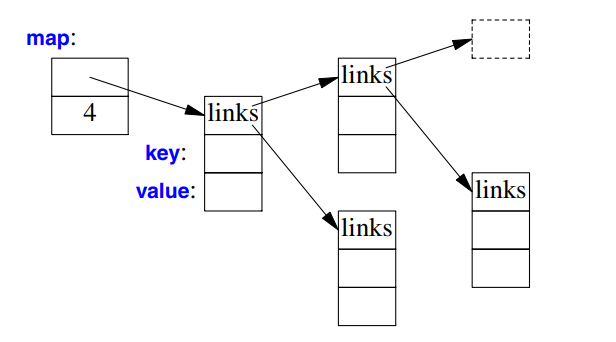In other contexts, a map is known as an associative array or a dictionary 🌺. It is implemented as a balanced binary tree 🌹

The standard-library map is a container of pairs of values optimized for lookup. We can use the same initializer as for `vector` and `list`.

No two mapped values can have same key values.

``````map<string,int> phone_book {
{"David Hume",123456},
{"Karl Popper",234567},
{"Ber trand Ar thur William Russell",345678}
};
``````

When indexed by a value of its first type (called the key), a map returns the corresponding value of the second type (called the value or the mapped type).

``````int get_number(const string& s)
{
return phone_book[s];
}
``````

The cost of a map lookup is `O(log(n))` where n is the number of elements in the map.. It is because the internal implementation of map is done using a balanced binary tree (Red-Black Tree) :punch: :punch:

### Basic functions of map

• `begin()` – Returns an iterator to the first element in the map
• `end()` – Returns an iterator to the theoretical element that follows last element in the map
• `size()` – Returns the number of elements in the map
• `max_size()` – Returns the maximum number of elements that the map can hold
• `empty()` – Returns whether the map is empty
• `insert(keyvalue, mapvalue)` – Adds a new element to the map
• `erase(iterator position)` – Removes the element at the position pointed by the iterator
• `erase(const g)`– Removes the key value ‘g’ from the map
• `clear()` – Removes all the elements from the ma
• `find()` is used to search for the key-value pair and accepts the “key” in its argument to find it. This function returns the pointer to the element if the element is found:punch: :punch:, else it returns the pointer pointing to the last position of map i.e “map.end()” .

### map vs unordered_map

• When it comes to efficiency, there is a huge difference between maps and unordered maps.

description map unordered_map
Ordering increasing order (by default) no ordering
Implementation Self balancing BST like Red-Black Tree Hash Table
search time log(n) O(1) -> Average O(n) -> Worst Case
Insertion time log(n) + Rebalance Same as search
Deletion time log(n) + Rebalance Same as search

### use std::map when :punch: :punch:

• You need ordered data.
• You would have to print/access the data (in sorted order).
• You need predecessor/successor of elements.

### Use std::unordered_map when

• You need to keep count of some data (Example – strings) and no ordering is required.
• You need single element access i.e. no traversal

### find() and count() function

• Count elements with a specific key searches the container for elements whose key is `k` and returns the `number of elements found`. Because unordered_map/map containers do not allow for duplicate keys, this means that the function actually returns `1` if an element with that key exists in the container, and zero otherwise.

### unordered_map vs unordered_set :

In unordered_set, we have only key, no value, these are mainly used to see presence/absence in a set. For example, consider the problem of counting frequencies of individual words. We can’t use unordered_set (or set) as we can’t store counts.

### how to retrieve all key-values from map

• Using Iterator
``````std::map<int, int> m;
std::vector<int> key, value;
for(std::map<int,int>::iterator it = m.begin(); it != m.end(); ++it) {
key.push_back(it->first);
value.push_back(it->second);
std::cout << "Key: " << it->first << std::endl();
std::cout << "Value: " << it->second << std::endl();
}
``````
``````* Using for-each statement
```c++
std::map<int,int> mapints;
std::vector<int> vints;
for(auto const& imap: mapints)
vints.push_back(imap.first);
``````

### std::map<Key,T,Compare,Allocator>::emplace

Inserts a new element into the container constructed in-place with the given args if there is no element with the key in the container

### C++ equivalent to Java Map getOrDefault?

• std::unordered_map::operator[]
• `mapped_type& operator[] ( const key_type& k );`
• If `k` matches the key of an element in the container, the function returns a reference to its mapped value.
• If `k` does not match the key of any element in the container, the function inserts a new element with that key and returns a reference to its mapped value. 👊 🔥 Notice that this always increases the container size by one, even if no mapped value is assigned to the element (the element is constructed using its default constructor) 🌺 🍀
• this is very important operator if you want to create a default values if key doesn't exist and use the existing values if key exists.

### Advantages of BST over Hash Table

• Hash Table supports following operations in Θ(1) time.
1. Search
2. Insert
3. Delete
• The time complexity of above operations in a self-balancing Binary Search Tree (BST) (like Red-Black Tree, AVL Tree, Splay Tree, etc) is `O(Logn)`.

• So Hash Table seems to beating BST in all common operations. When should we prefer BST over Hash Tables

• Its because of the following reasons

• We can get all keys in sorted order by just doing In-order Traversal BST. This is not a natural operation in Hash Tables and requires extra efforts.
• Doing order statistics, finding closest lower and greater elements, doing range queries are easy to do with BSTs. Like sorting, these operations are not a natural operation with Hash Tables
• BSTs are easy to implement compared to hashing, we can easily implement our own customized BST. To implement Hashing, we generally rely on libraries provided by programming languages.